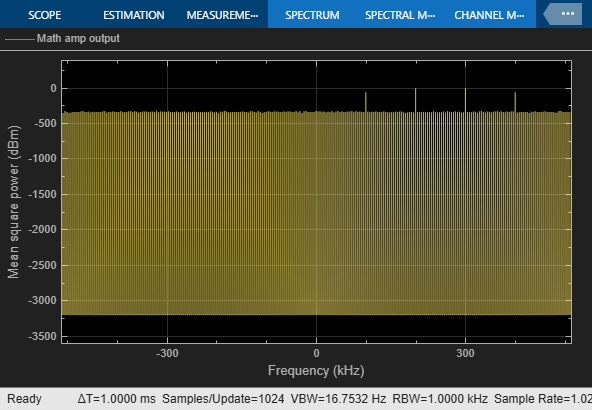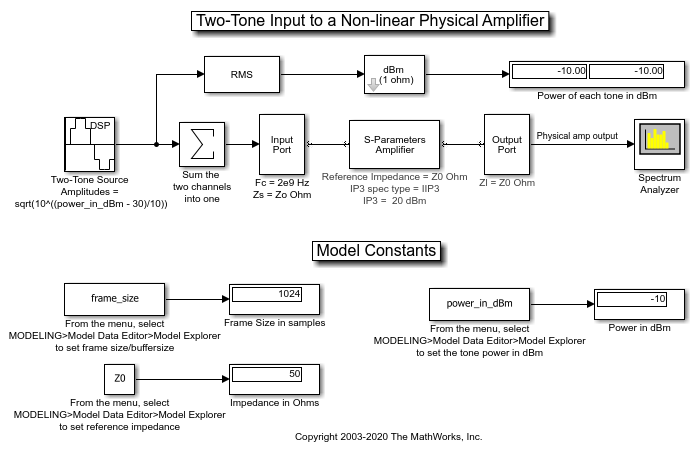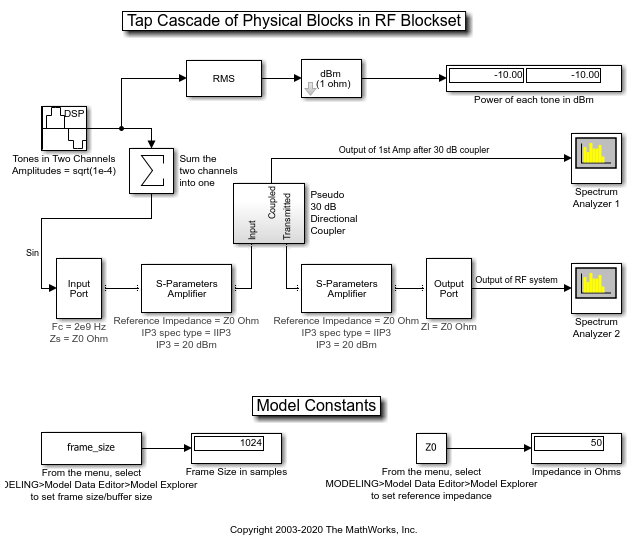# Power in Simulink Sources and Signals

This example shows how to use the Input Port and Output Port blocks of the RF Blockset™ Equivalent Baseband library to convert between dimensionless Simulink® signals and equivalent-baseband signals.

In general, signals in Simulink are dimensionless, so their amplitudes do not correspond to a particular voltage or power. However, in an RF system, power is a quantity of interest. When you use blocks from the RF Blockset Equivalent Baseband library in a Simulink model, you must specify how the software interprets the Simulink signals that exist outside the boundaries of the Input Port and Output Port blocks. RF Blockset Equivalent Baseband software provides two options to interpret the Simulink signal: power wave or voltage. The amplitude of a source in Simulink determines the signal power level and affects the signal power and power spectrum.

All the models used in this example interpret the Simulink signal as a power wave with dimensions of. This means for an RF system, the source signal generated by regular Simulink blocks is treated as the incident power wave to the RF system, and the RF output signal is the transmitted power wave of the RF system. If you choose to interpret the Simulink signal as a voltage, you need to modify the models by considering the impedance effects when you calculate the powers. For more details, see Convert to and from Simulink Signals.

### White Noise Source

This part of the example shows how to create a complex baseband-equivalent White Noise Source. This type of source is useful, for example, as a stimulus for visualizing the frequency response of an RF system. Use a Random Source block from the DSP System Toolbox™ Sources sublibrary to create this source. In the Random Source block dialog box, set the Complexity parameter to `Complex` and in the Variance parameter enter the desired noise power in watts using the expression `power_in_watts`.To calculate a signal power in dBm, use an RMS block (from the DSP System Toolbox Statistics sublibrary), followed by a dB Conversion block (from the DSP System Toolbox Math Functions/Math Operations sublibrary). In the dB Conversion block dialog box, set Convert to parameter to `dBm` , Input Signal parameter to `Amplitude` , and Load resistance (ohms) parameter to `1` .

To display the power spectrum of a signal, use the Spectrum Analyzer block (from the RF Blockset Circuit Envelope Utilities sublibrary). To show the Spectrum Settings, in the Spectrum Analyzer menu, select View > Spectrum Settings or use the far left button in the toolbar. Two-sided spectrum is checked by default in the trace options. This is the desired frequency range because a complex baseband-equivalent representation translates the carrier frequency to zero hertz. The real-world frequencies above and below the carrier (i.e. higher and lower sidebands) are represented as positive and negative frequencies, respectively. A few of the Spectrum Settings options (Type, Window, Units, Averaging method, Averages) have been changed from their default.In addition, note that the selected Spectrum Scope Window Type can affect how the power is distributed among the channels closest to the actual frequency. For example, if a pure sine wave falls between two channels, you may need to sum the power in one or two channels either side of the actual frequency to determine the exact total power.

### Complex Sine Source

The next model, of a Complex Sine Wave, shows how to use power to set the amplitude of a complex sine wave source block for an RF system. Complex sine wave sources are often used in baseband-equivalent Simulink models. These sources have the following time-domain output:

`signal(t) = amplitude * (cos(2*pi*f*t+phi)+j*sin(2*pi*f*t+phi))`

The mean square power of the output, `signal`, is `amplitude^2`.

By contrast, the time-domain output of a real sine wave source is:

`signal2(t) = amplitude * sin(2*pi*f*t+phi)`

where the mean square power of `signal2` is `amplitude^2/2`, half that of a complex sine wave with the same `amplitude`.

Use the Sine Wave block to create a complex sine source. In the block dialog box, set the Output complexity parameter to `Complex` and the Amplitude parameter to `sqrt(power_in_watts)`. By default, the Spectrum Analyzer displays power spectral density normalized to the unit sampling frequency in units of dBm/Hertz. For this section, the Spectrum Scope displays the tone as a positive frequency (upper side band).### Two-Tone Input to Idealized Baseband Nonlinear Amplifier

The third model, of a Two-Tone Input to an Idealized Baseband Nonlinear Amplifier, shows how the Amplifier block in the RF Blockset Idealized Baseband library affects the signal. In the Amplifier block dialog box set the IIP3 (dBm) parameter to `20` dBm. In the Sine Wave block dialog box, set Amplitude parameter to `sqrt(10^((power_in_dBm - 30)/10))`. Setting `power_in_dBm = -10` in the model workspace results in -10 dBm per tone. Note that we must use a Matrix Sum block with the Sum along parameter set to "Rows" after the source block to sum the two-channel output of the source. Without the Row Sum, a two-channel signal would be created, all blocks downstream would have two independent channels, and no mixing would occur.The Spectrum Scope displays the power level in each intermodulation tone. The power level of each is:

`-10dBm - 2*(20dBm - -10dBm) = -70dBm`.

### Two-Tone Input to Equivalent Baseband Nonlinear Amplifier

Like the third model, the fourth model, of a Two-Tone Input to an Equivalent Baseband Nonlinear Amplifier, shows how an Amplifier block affects the signal. However, this time we use the S-Parameters Amplifier block from the Equivalent Baseband library of RF Blockset. Unlike the Idealized Baseband blocks, the Equivalent Baseband blocks allow you to set the center frequency and impedances. Thus, if you want to model an RF system at real RF frequencies, the loading and reflection effects, we recommend these physical blocks.

In this model, we set several parameters to `Z0`:

• Source impedance parameter of the Input Port block dialog box

• Reference impedance parameter of the S-Parameters Amplifier block dialog box

• Load impedance parameter of the Output Port block dialog box

In the Input Port block, set the Center frequency (Hz) parameter to `2e9` (2 GHz). The baseband frequencies of the two-tone complex Simulink signal are 200 kHz and 300 kHz. Thus, in the RF system (the Equivalent Baseband blocks connected between the Input Port and Output Port blocks), the real RF two-tone frequencies are 2.0002 GHz and 2.0003 GHz. By default, the Spectrum Scope displays in baseband. To display the desired tones at 2.0002 GHz and 2.0003 GHz (-10 dBm each) and the intermodulation tones at 2.0001 GHz and 2.0004 GHz (-70 dBm each), set the Frequency display offset: parameter in the "Axis Properties" tab to the value of the Center frequency, in this case it is 2e9 (2 GHz).### Displaying Power Spectrum Within Cascade of RF Blockset Equivalent Baseband Blocks

The Output Port block lets you create a link budget plot for multi-block cascades. This feature allows you to visualize the characteristics of the cascade non-intrusively. Therefore, it is not usually necessary to tap a cascade of RF Blockset Equivalent Baseband blocks. However, it is sometimes useful to do so, for example, to see the modulated spectrum at an intermediate point. The final model of a Tap Cascade of Equivalent Baseband Blocks in RF Blockset, achieves this with a subsystem that approximately models a real-world directional coupler. As with its real-world counterpart, the tapping is intrusive in that it presents a load impedance to the downstream part of the cascade and it drives the upstream part with a source impedance.

Double click on the subsystem "Pseudo 30 dB Directional Coupler" to open it and see how the model works. The Output Port and Input Port blocks correspond to the input and output impedance of the mainline of a real-world directional coupler, respectively. However, the phase behavior of a real-world directional coupler is not modeled here.The first Spectrum Scope shows the intermodulation tones after one amplifier. Note that the power is 30 dB down because of the characteristics of the "Pseudo 30 dB Directional Coupler" subsystem. You could calibrate this out with a gain block, or even modify the subsystem to model a 0 dB-loss "active" directional coupler. The second Spectrum Scope shows an increased level of intermodulation tones after a cascade of two non-linear amplifiers. The second Spectrum Scope shows an increased level of intermodulation tones after a cascade of two non-linear amplifiers.